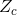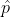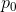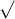Question

# You wish to test the following claim (H a) at a significance level of α = 0.02.

You wish to test the following claim (${H}_{a}$) at a significance level of $\alpha =0.02$.

${H}_{o}:{p}_{1}={p}_{2}$
${H}_{a}:{p}_{1}<{p}_{2}$

You obtain 380 successes in a sample of size ${n}_{1}=641$ from the first population. You obtain 179 successes in a sample of size ${n}_{2}=259$ from the second population. For this test, you should NOT use the continuity correction, and you should use the normal distribution as an approximation for the binomial distribution.

What is the test statistic for this sample? (Report answer accurate to three decimal places.)
test statistic =

What is the p-value for this sample? (Report answer accurate to four decimal places.)
p-value =

The p-value is...

This test statistic leads to a decision to...

As such, the final conclusion is that...

Solution :

Given that,

This a left (One) tailed test.

Critical value of  the significance level is α = 0.02, and the critical value for a two-tailed test is= -2.054= x / n = 423 / 558 = 0.76

Test statistics

z = (-) /*(1-) / n

= ( 0.76 - 0.77) /(0.77*0.23) / 558

-0.561

#### Earn Coins

Coins can be redeemed for fabulous gifts.

Similar Homework Help Questions
• ### You wish to test the following claim (H1H1) at a significance level of α=0.02α=0.02.       Ho:p1=p2Ho:p1=p2       H1:p1<p2H1:p1<p2...

You wish to test the following claim (H1H1) at a significance level of α=0.02α=0.02.       Ho:p1=p2Ho:p1=p2       H1:p1<p2H1:p1<p2 You obtain 33.7% successes in a sample of size n1=718n1=718 from the first population. You obtain 42.5% successes in a sample of size n2=414n2=414 from the second population. What is the test statistic for this sample? (Report answer accurate to three decimal places.) test statistic = What is the p-value for this sample? (Report answer accurate to four decimal places.) p-value = The p-value...

• ### You wish to test the following claim ( H a H a ) at a significance level of α = 0.10 α = 0.10 . H...

You wish to test the following claim ( H a H a ) at a significance level of α = 0.10 α = 0.10 . H o : p 1 = p 2 H o : p 1 = p 2 H a : p 1 > p 2 H a : p 1 > p 2 You obtain 432 successes in a sample of size n 1 = 681 n 1 = 681 from the first population. You obtain...

• ### You wish to test the following claim (HaHa) at a significance level of α=0.10       Ho:p1=p2       Ha:p1>p2...

You wish to test the following claim (HaHa) at a significance level of α=0.10       Ho:p1=p2       Ha:p1>p2 You obtain 37 successes in a sample of size 616 from the first population. You obtain 19 successes in a sample of size 603 from the second population. What is the test statistic for this sample? (Report answer accurate to three decimal places.) Test statistic = What is the p-value for this sample? (Report answer accurate to four decimal places.) p-value = The p-value...

• ### You wish to test the following claim ( H a ) at a significance level of...

You wish to test the following claim ( H a ) at a significance level of α = 0.005 . H o : p 1 = p 2 H a : p 1 < p 2 You obtain 11.3% successes in a sample of size n 1 = 781 from the first population. You obtain 14.2% successes in a sample of size n 2 = 639 from the second population. For this test, use the normal distribution as an approximation...

• ### You wish to test the following claim (Ha) at a significance level of α=0.10.       Ho:p1=p2 &n...

You wish to test the following claim (Ha) at a significance level of α=0.10.       Ho:p1=p2       Ha:p1<p2 You obtain 13.3% successes in a sample of size n1=226 from the first population. You obtain 21.4% successes in a sample of size n2=541 from the second population. For this test, you should NOT use the continuity correction, and you should use the normal distribution as an approximation for the binomial distribution. What is the test statistic for this sample? (Report answer...

• ### You wish to test the following claim (Ha) at a significance level of α=0.005.       Ho:p1=p2...

You wish to test the following claim (Ha) at a significance level of α=0.005.       Ho:p1=p2       Ha:p1<p2 You obtain 23.9% successes in a sample of size n1=201 from the first population. You obtain 31.7% successes in a sample of size n2=514 from the second population. For this test, you should NOT use the continuity correction, and you should use the normal distribution as an approximation for the binomial distribution. What is the critical value for this test? (Report answer...

• ### You wish to test the following claim (H1) at a significance level of α=0.10.       Ho:p1=p2       H1:p1<p2...

You wish to test the following claim (H1) at a significance level of α=0.10.       Ho:p1=p2       H1:p1<p2 You obtain a sample from the first population with 423 successes and 41 failures. You obtain a sample from the second population with 294 successes and 25 failures. What is the critical value for this test? (Report answer accurate to three decimal places.) critical value = What is the test statistic for this sample? (Report answer accurate to three decimal places.) test statistic =...

• ### You wish to test the following claim (HaHa) at a significance level of α=0.01α=0.01.       Ho:p1=p2Ho:p1=p2       Ha:p1>p2Ha:p1>p2...

You wish to test the following claim (HaHa) at a significance level of α=0.01α=0.01.       Ho:p1=p2Ho:p1=p2       Ha:p1>p2Ha:p1>p2 You obtain 212 successes in a sample of size 450 from the first population. You obtain 74 successes in a sample of size 226 from the second population. What is the test statistic for this sample? (Report answer accurate to three decimal places.) Test statistic = What is the p-value for this sample? (Report answer accurate to four decimal places.) p-value = The p-value...

• ### You wish to test the following claim (HaHa) at a significance level of α=0.002α=0.002.       Ho:p1=p2Ho:p1=p2       Ha:p1<p2Ha:p1<p2...

You wish to test the following claim (HaHa) at a significance level of α=0.002α=0.002.       Ho:p1=p2Ho:p1=p2       Ha:p1<p2Ha:p1<p2 You obtain 19.6% successes in a sample of size n1=588n1=588 from the first population. You obtain 26.4% successes in a sample of size n2=277n2=277from the second population. For this test, you should NOT use the continuity correction, and you should use the normal distribution as an approximation for the binomial distribution. What is the test statistic for this sample? (Report answer accurate to three...

• ### You wish to test the following claim (Ha Ha ) at a significance level of α=0.05...

You wish to test the following claim (Ha Ha ) at a significance level of α=0.05 α=0.05 . Ho:p1=p2 Ho:p1=p2 Ha:p1>p2 Ha:p1>p2 You obtain 82.6% successes in a sample of size n1=447 n1=447 from the first population. You obtain 75.2% successes in a sample of size n2=472 n2=472 from the second population. For this test, you should NOT use the continuity correction, and you should use the normal distribution as an approximation for the binomial distribution. The test statistic's value...